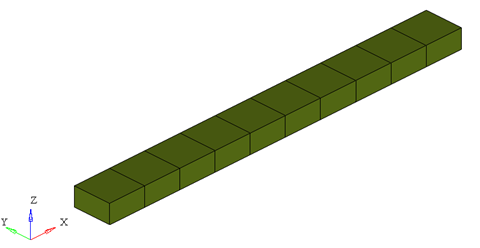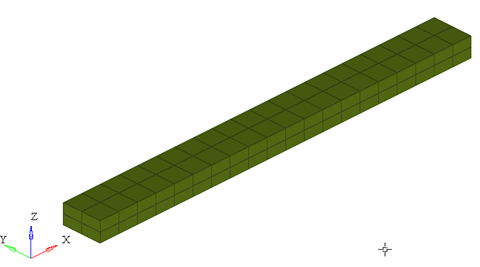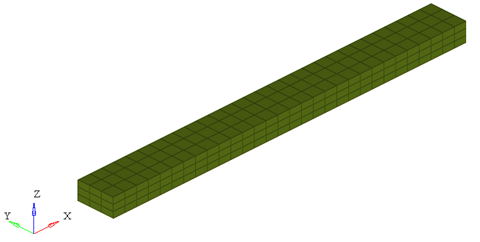# PBEAM9

Geometric Properties ElementPBEAM9 lets you specify the geometric properties for an associated beam element of an arbitrary cross-section using a polynomial expression

## Format

<PBEAM9
id       = "integer"
mid      = "integer"
w1       = "real"
w2       = "real"
nf       = "real"
nx       = "integer"
ny       = "integer"
nz       = "integer"
ngx      = "integer"
ngy      = "integer"
ngz      = "integer"
a0       = "real"
...
a6       = "real"
b0       = "real"
...
b6       = "real"
graph    = "integer"
/>

## Attributes

id
Unique beam property identification number.
mid
Material property identification number.
w1
Left limit of the polynomial functions.
w2
Right limit of the polynomial functions.
nf
This attribute lets you specify a number that is used to reduce the bending stiffness.
The default for nf is 1.
nx, ny, nz
Number of integration points in X, Y and Z directions.
Default for nx is 5, ny is 3 and nz is 3.
ngx, ngy, ngz
Number of sub elements in X, Y and Z directions. The default for all three is 1. 4.
a0,a1,…a6
The coefficients of the 6th order polynomial function that is used to represent the upper limit of the cross section.
b0,b1,…b6
The coefficients of the 6th order polynomial function that is used to represent the lower limit of the cross section.
graph
A post-processing flag that determines how this element is represented in the animation H3D. Default is 2.

## Example

The example demonstrates the definition of a PBEAM9 property element.

<PBEAM9 id="1" mid="1" w1="-50." w2="50." a0="50." b0="-20" nf="1" ngx="5" ngy="3" ngz="3" />

1. This type of property card is used to specify the geometric properties of the BEAM9 element. Each beam property element must have a unique identification number.
2. This property card defines the geometrical properties of the beam. The material properties of the beam are defined by the material specified by mid.
3. The coefficients a0-a6 and b1-b6 represent the coefficients of the upper and lower limits of the cross section as shown in Figure 1 below.

4. graph is a post processing flag that determines how this element will be represented in the animation H3D file.
• graph = "0" implies that this element will not be represented in the H3D
• graph = "1" implies that this element will be represented as a line drawn between the two connecting nodes.
Note: When using graph="0" or graph="1", you will not be able to visualize the stress, strain or displacement contours. To do this, use graph="2" or graph="3".
• graph = "2" implies that the beam will be represented by 3D solid elements. This mode is useful when trying to visualize the stress/strain and displacement contours.
• graph = "3" implies that the beam is represented both as 3D solid elements as well as a line connecting the two nodes of the beam. This is useful when you need to visualize both the center line and the 3D representation of the beam.

When representing the beam as a solid, the arguments ngx, ngy and ngz determine the number of elements that are used to represent the 3D beam in the animation H3D.ngx = ngy = ngz = 1ngx = ngy = ngz = 2ngx = ngy = ngz = 3

While increasing the ngx, ngy and ngz results in a better representation of the beam, it also increases the post-processing time taken by MotionSolve to write out the H3D. In addition, large values of ngx, ngy and ngz will increase the file size of the H3D considerably. Consider using the minimum values of these attributes that satisfy your visualization needs.